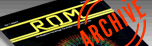`ROM MAGAZINE ISSUE 6 — JUNE/JULY 1984 / PAGE 46`

 THE COVER by Bob Cockroft     The following is the program that produced the art on the front cover. The entire design was drawn on a graphics 8 screen. The various colours were created by using two methods: artifacting, and adjusting the colour controls on the Television. The distant spaceship was drawn and coloured completely by artifacting. If examined on a non-colour screen, its shape would be seen as a series of vertical lines. By plotting either even or odd pixels, different colours can be created. A similar, but less dramatic effect can be seen with the overlapping circles in the Atari Symbol. The varying background colour is not created with the computer. By experimenting with our televisions colour controls, we were able to get the screen to do some strange things. Multicoloured screens and other bazaar effects were seen and photographed. As the effects got stranger, we tried even more to disturb the televisions normal colour patterns. When it was all over, we had a number of interesting photographs. Although our television may never again work the same, we felt we had a cover for the sixth issue. After much discussion, we finally decided to use the photograph you see on the front cover.     The most mathematically involved part of the cover is the Atari symbol. The circles were created by using the following formula. x^2 +y^2 =r ^2     The columns of circles were molded into an Atari symbol by three vertical lines. The two outside lines were exponential so that they curved upwards. y=x^2     The center of the circles were placed on each of these lines. The lines were then erased to reveal the shape you see. 2 P=1 4 RDU=20 5 F=85 6 CT=0:RAT=15:RAT1=RAT 7 B=130 8 C=130 10 GRAPHICS 8 20 COLOR 0 30 SETCOLOR 2,16,1 40 XP=60:YP=B 50 X=0:Y=0 100 PLOT XP+X,YP+Y 105 IF CT=0 THEN RDU=RDU-1:GOTO 400 110 X=X+1:CT=CT+1 120 Y=-1/C*(X*X) 130 RAT1=RAT1-0.155:IF CT>RAT1 THEN CT=0 140 IF X>F THEN FIN=YP+Y:GOTO 200 150 GOTO 100 199 REM * DRAW ATARI SYMBOL * 200 XP=265:YP=B:CT=0:P=2:RAT=15:RAT1=RAT:RDU=20 205 XP1=265 210 X=0:Y=0 220 PLOT XP+X,YP+Y 224 IF CT=0 THEN RDU=RDU-1:GOTO 400 230 X=X-1:CT=CT+1 240 Y=-1/C*(X*X) 250 IF X<-1*F THEN 300 255 RAT1=RAT1-0.155:IF CT>RAT1 THEN CT=0 260 GOTO 220 300 XP=INT((60+265)/2)+1:P=3:RAT=12:RAT1=RAT:RDU=20 305 X=0:Y=0:YP=B:CT=0 310 PLOT XP,YP+Y 312 IF CT=0 THEN RDU=RDU-1.45:GOT0 400 314 Y=Y-1:CT=CT+1 315 RAT1=RAT1-0.15:IF CT>RAT1 THEN CT=0 317 IF YP+Y